Question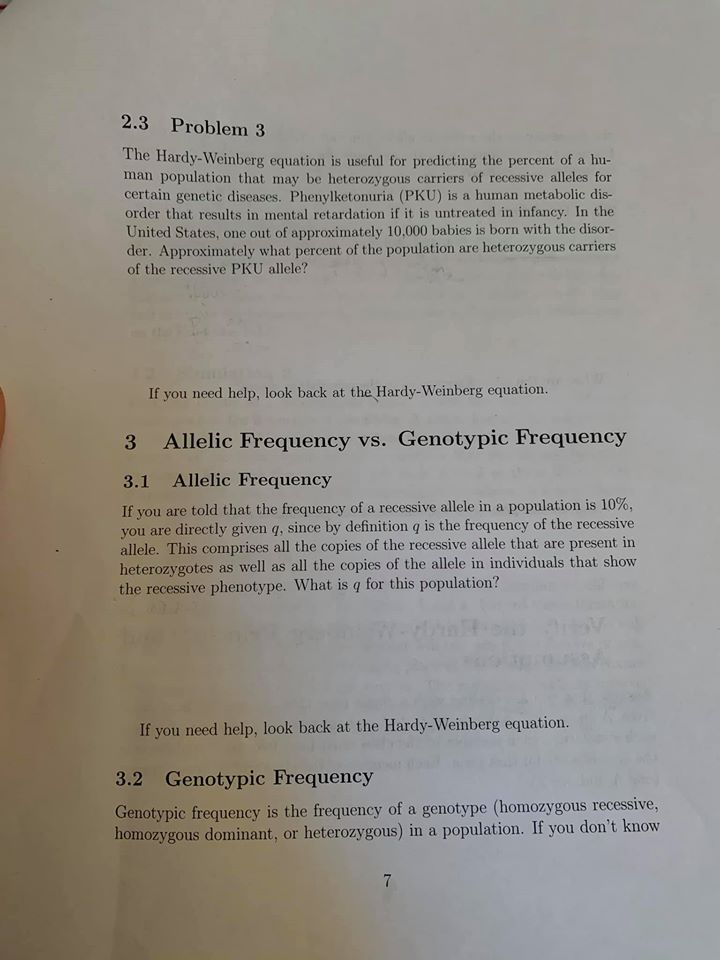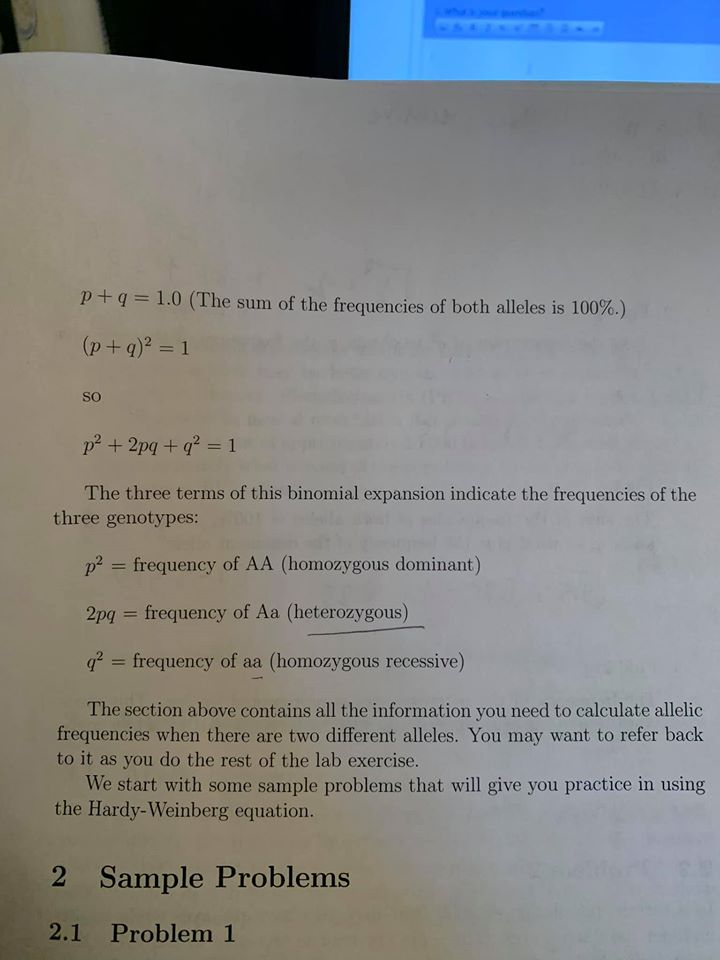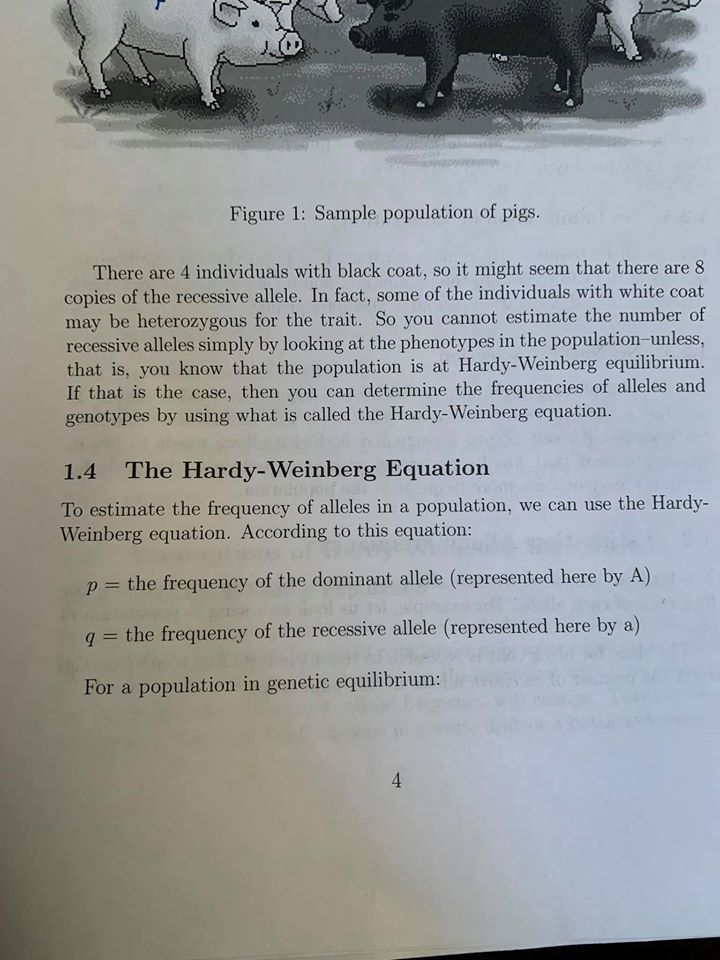Hardy-Weinberg equilibrium:

· Hardy-Weinberg equation describes: The most possible distribution of the population genotype of the population, if the frequencies of the alleles are known.

· If allele frequencies are p and q, then the genotype frequencies are p2, q2, 2pq.

· A population is considered to be in Hardy-Weinberg equilibrium when: In a randomly mating population, allele frequency and genotype frequency remain constant.

· Thus, under Hardy-Weinberg equilibrium:

p+ q=1 and

p2+2pq + q2 = 1.

Problem 3.

Representation:

• Genotype for individuals with PKU = pp = recessive homozygous condition.
• Normal individuals, will have genotypes:
• PP = Homozygous dominant
• Pp = Heterozygous dominant (Carriers)
• Since 1 out of 10,000 babies in the population have PKU = pp
• Genotype frequency for pp = q2 = 1/ 10000 = 0.0001
• Allele frequency = q = 0.01
• As per Hardy-Weinberg equilibrium p+q = 1
• Thus, p = 1- 0.01 = 0.99
• Genotype frequency for PP = p2= (0.99)2= 0.9801
• Heterozygous genotype frequency (Pp) = 2 x p x q =2 x 0.01x 0.99 =0.0198

#### Earn Coins

Coins can be redeemed for fabulous gifts.

Similar Homework Help Questions
• ### please click on the photo to see all of it The basic equations of Hardy-Weinberg Equilibrium...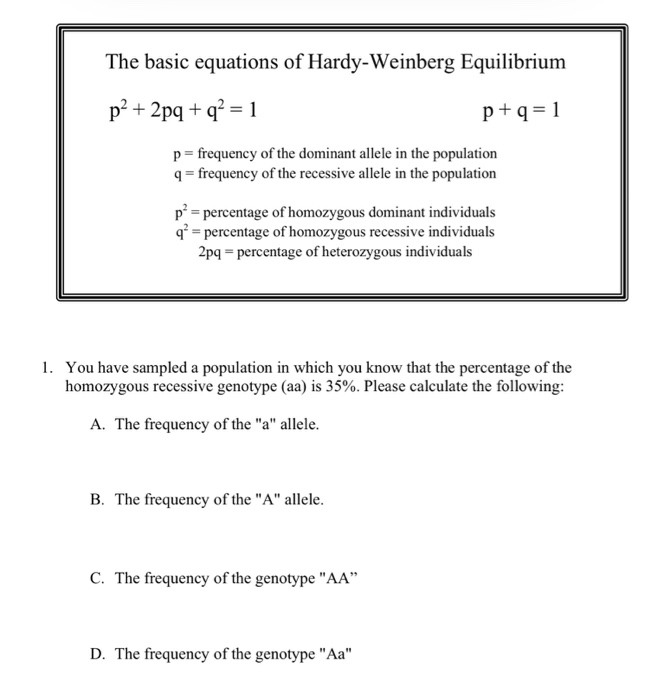please click on the photo to see all of it The basic equations of Hardy-Weinberg Equilibrium p² + 2pq + q2 = 1 p+q=1 p= frequency of the dominant allele in the population 9 = frequency of the recessive allele in the population př= percentage of homozygous dominant individuals q* = percentage of homozygous recessive individuals 2pq - percentage of heterozygous individuals 1. You have sampled a population in which you know that the percentage of the homozygous recessive genotype...

• ### Hardy Weinberg assignment P + Q = 1 In which P represents frequency of dominant allele...

Hardy Weinberg assignment P + Q = 1 In which P represents frequency of dominant allele and Q represents frequency of the recessive allele P2 + 2PQ + Q2 =1 P2 represents frequency of homozygous dominant 2PQ represents frequency of heterozygous Q2 represents frequency of homozygous recessive Consider a population of beetles on an island. There are 1000 beetles and they have different colored wings. Black wings are dominant over silver wings. Calculate the allele and the genotypic frequencies in...

• ### The Hardy-Weinberg principle and its equations predict that frequencies of alleles and genotypes remain constant from generation to generation in populations that are not evolving

.1. The Hardy-Weinberg principle and its equations predict that frequencies of alleles and genotypes remain constant from generation to generation in populations that are not evolving. What five conditions does this prediction assume to be true about such a population? a._______  b._______  c._______  d._______  e._______  2. Before beginning the activity, answer the following general Hardy-Weinberg problems for practice (assume that the population is at Hardy-Weinberg equilibrium).a. If the frequency of a recessive allele is 0.3, what is the frequency of the dominant...

• ### Consider a locus of interest that has two alleles: A and a. A diploid individual carrying...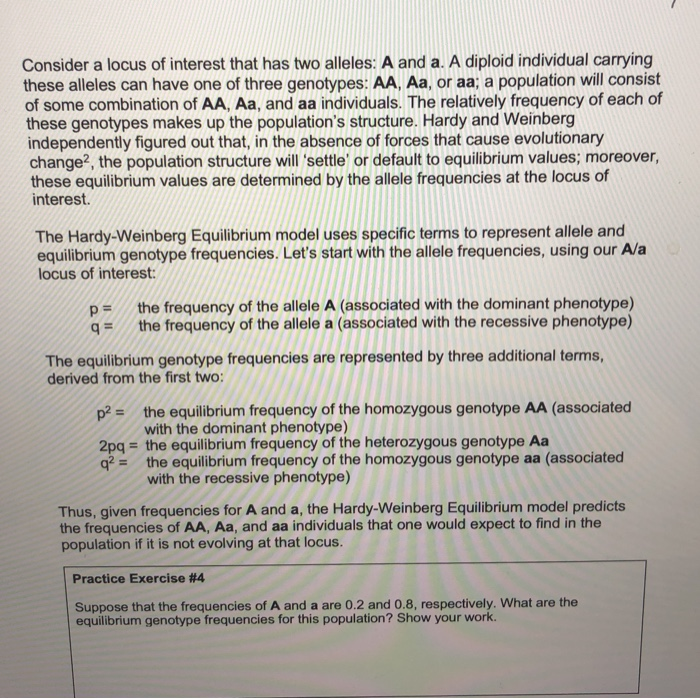Consider a locus of interest that has two alleles: A and a. A diploid individual carrying these alleles can have one of three genotypes: AA, Aa, or aa; a population will consist of some combination of AA, Aa, and aa individuals. The relatively frequency of each of these genotypes makes up the population's structure. Hardy and Weinberg independently figured out that, in the absence of forces that cause evolutionary change, the population structure will 'settle' or default to equilibrium values,...

• ### Use the Hardy-Weinberg equations (p2 + 2pq + q2 = 1 and p + q =...

Use the Hardy-Weinberg equations (p2 + 2pq + q2 = 1 and p + q = 1) to find the genotypes of the following hypothetical phenotypic information: a.       Within a particular population, you observe that 450 individuals have freckles (dominant, F) and 550 do not have freckles (recessive, f). b.      Homozygous dominant (frequency) ______(A)_________ c.       Heterozygous (frequency) ______(B)_____________ d.      Homozygous recessive (frequency) _______(C)____________

• ### EXERCISE 6 HARDY-WEINBERG EQUILIBRIUM Work in a small group or alone to complete this exercise. In...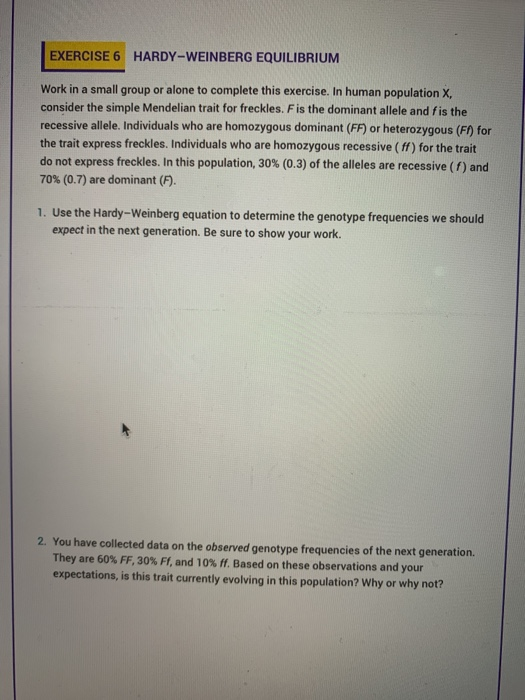EXERCISE 6 HARDY-WEINBERG EQUILIBRIUM Work in a small group or alone to complete this exercise. In human population X consider the simple Mendelian trait for freckles. F is the dominant allele and fis the recessive allele. Individuals who are homozygous dominant (FF) or heterozygous (F) for the trait express freckles. Individuals who are homozygous recessive (ff) for the trait do not express freckles. In this population, 30% (0.3) of the alleles are recessive (1) and 70% (0.7) are dominant (F)....

• ### Calculating expected genotypic frequencies and individuals in a population from allele frequencies: 3.1 In a population...

Calculating expected genotypic frequencies and individuals in a population from allele frequencies: 3.1 In a population of peas, the frequency of the dominant allele for a purple flower is 0.77 and the frequency of the recessive allele for a white flower is 0.23. What would the genotypic frequencies be if the population is in equilibrium? _____   = Frequency of homozygous dominant individuals _____   = Frequency of heterozygous individuals _____   = Frequency of homozygous recessive individuals How many individuals would you...

• ### Hardy-Weinberg Practice Problems: You need to list equations used and provide steps of problem solving. Providing...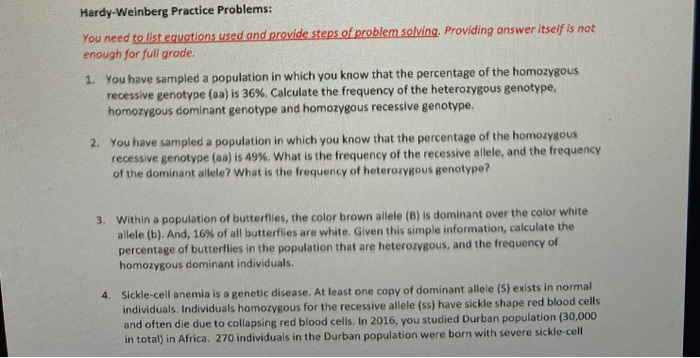Hardy-Weinberg Practice Problems: You need to list equations used and provide steps of problem solving. Providing answer itself is not enough for full grade. 1. You have sampled a population in which you know that the percentage of the homozygous recessive genotype (aa) is 36%. Calculate the frequency of the heterozygous genotype, homozygous dominant genotype and homozygous recessive genotype. 2. You have sampled a population in which you know that the percentage of the homozygous recessive genotype (aa) is 49%....

• ### Give the Hardy–Weinberg expected genotypic frequencies for an autosomal locus with three alleles, where the frequencies...

Give the Hardy–Weinberg expected genotypic frequencies for an autosomal locus with three alleles, where the frequencies of alleles A1, A2, and A3 are defined as p, q, and r, respectively. Set the problem up and show your work. Tay–Sachs disease is an autosomal recessive disorder. Among Ashkenazi Jews, the frequency of Tay–Sachs disease is 1 in 3600. If the Ashkenazi population is mating randomly for the Tay–Sachs gene, what proportion of the population consists of heterozygous carriers of the Tay–Sachs...

• ### Q3 Question 3 1 Point In a Hardy-Weinberg situation, suppose that cystic fibrosis (a rare genetic...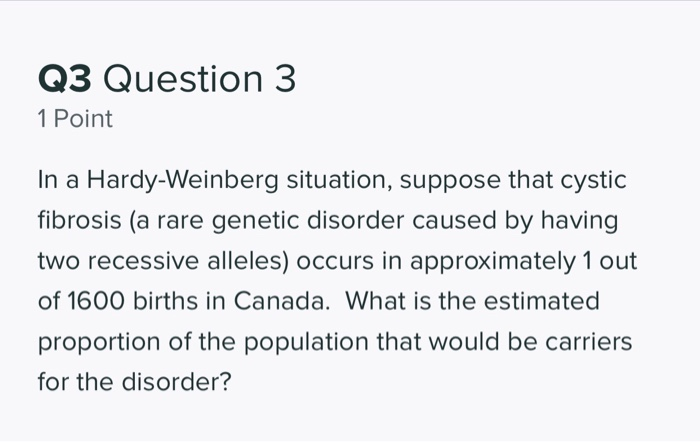Q3 Question 3 1 Point In a Hardy-Weinberg situation, suppose that cystic fibrosis (a rare genetic disorder caused by having two recessive alleles) occurs in approximately 1 out of 1600 births in Canada. What is the estimated proportion of the population that would be carriers for the disorder? Q4 Question 4 2 Points For some genetically linked disorders, the survival of the heterozygous individual may be less than that of a homozygous dominant individual (while homozygous recessive individuals still have...